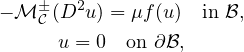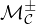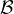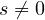Electron. J. Differential Equations, Vol. 2021 (2021), No. 31, pp. 1-19.

### Existence and multiplicity for radially symmetric solutions to Hamilton-Jacobi-Bellman equations Xiaoyan Li, Bian-Xia Yang

Abstract:whereare general Hamilton-Jacobi-Bellman operators, μ is a real parameter andis the unit ball. By using bifurcation theory, we determine the range of parameter μ in which the above problem has one or multiple nodal solutions according to the behavior of f at 0 and infinity, and whether f satisfies the signum condition f(s)s>0 foror not.Xiaoyan Li Department of Mathematics and Statistics Northwest Normal University Lanzhou, Gansu 730000, China email: 17242502@qq.com Bian-Xia Yang College of Science Northwest A&F University Yangling, Shaanxi 712100, China email: yanglina7765309@163.com